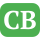CppBuzz.com

# C++ program to find avg of top 4 score

```#include<iostream>
using namespace std;

void getScore(float &score)
{
cout<<"\n Enter test score : ";
cin>>score;
}

float  findLowest(float arr[])
{
float lowest = arr;

for(int i = 1 ; i<5; i++)
{
if(lowest>arr[i])
{
lowest = arr[i];
}
}

cout<<"\n Lowest is : "<<lowest;

return lowest;
}

void calcAverage(float arr[]){

float lowest = findLowest(arr);
float avg = 0;

for(int i = 0; i< 5; i++)
{
if(arr[i] !=  lowest)
avg = avg + arr[i];
}
avg = avg/4;
cout<<"\n Average is : "<< avg;
}

int main()
{
float arr;
for(int i = 0; i< 5; i++)
{
getScore(arr[i]);
}

calcAverage(arr);
return 0;
}
```